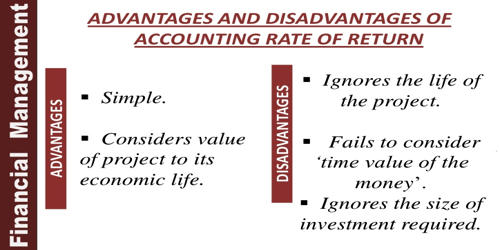Accounting

# Disadvantages of Accounting Rate of Return (ARR)The accounting rate of return (ARR) is a way of comparing the profits you expect to make from an investment to the amount you need to invest. The ARR is widely used to provide a rough guide to how attractive investment is. The main advantage is that it is easy to understand. The higher the ARR, the more attractive the investment is.

Disadvantages of Accounting Rate of Return (ARR)

(a) ARR ignores the time value of money. The primary weakness of the average return method of selecting alternative uses of funds is that the time value of funds is ignored.

(b) Unlike other methods of investment appraisal, the ARR is based on profits rather than cashflow. ARR method ignores the cash flow from the investment. If an investment quickly generates cash inflow, the company can invest in other profitable projects. But the accounting rate of return method focuses on accounting net operating income rather than cash flow. It is affected by subjective, non-cash items such as the rate of depreciation you use to calculate profits.

(c) There are also several different formulas that can be used to calculate an ARR. ARR method does not consider the terminal value of the project. If you use the ARR to compare different investments, you must be sure that you are calculating the ARR on a consistent basis.

(d) The ARR also fails to take into account the timing of profits. In calculating ARR, a £100,000 profit five years away is given just as much weight as a £100,000 profit next year. It does not take into consideration cash inflows which are more important than the accounting profits. In reality, you would prefer to get the profit sooner rather than later.

(e) This method does not consider the external factors which are also affecting the profitability of the project. The accounting rate of return does not remain constant over a useful life for many projects. This method cannot be applied in a situation when investing in a project to be made in parts. A project may, therefore, look desirable in one period but undesirable in another period.

(f) It is not useful to evaluate the projects where investment is made in two or more installments at different times. The results are different if one calculates ROI and others calculate ARR. It creates a problem in making decisions.

(g) The lifetime of multiple investments is not considered by the method. But while calculating the average earnings, the lifetime of investments are taken into account. It only considers the rate of return of the project but ignores the length of project lives